# Stumbling Into an Interesting Example (Inverse Functions)

I was working on preparing problems for precalculus class on inverse functions. I started to type the following run-of-the-mill problem type:

If f(x) = blah and g(x) = bleh, show f(x) and g(x) are inverse functions.

And the work usually goes something like this:

f(g(x)) = f(bleh) = algebra kung fu happens here = x
g(f(x)) = g(blah) = some more algebra kung fu = x
Then conclude f(x) and g(x) are inverses.

Without thinking about it, I typed f(x) = -x - 6. Then I wrote the statement on my pad of paper, started working... exchanged the y and x, solved for y.... and got the exact same function.

y = -x - 6. Hmmm.

A part of me wondered if I had made a careless error. Double checked. Nope. No error. I wondered if the graph of the function was its own reflection across the y = x line.Sure enough... Since y = -x - 6 is perpendicular to the line y=x, it will be its own reflection across the y=x line and consequently its own inverse. In fact, this made me think of an interesting question to pose to my students...

"Can you define a class of linear functions that are all their own inverses?"

In hindsight, perhaps I should be more mindful when constructing tasks for my students. But then again, this would be a great discussion to have with my students. Why does the function end up being its own inverse? Can we think of non-linear functions that are their own inverse? Can we define these classes of functions carefully?

# Combining Like Terms and Teaching Factoring

While lesson planning for calculus class, I was thinking this morning about common mistakes students make during simplification. Teaching students how to combine like terms can be a challenge. I'd like to share how I approach teaching combine like terms and factoring. Consider the Algebra 1 exercise below.

Textbook instructions:
"Simplify the following expressions. Use the Distributive Property if needed."

Problem: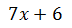How often do we see struggling students claim the above binomial is equivalent to 13x? We can quickly diagnose the misconception the student has... the student saw the addition symbol and combined the integers 7 and 6 as they did in their youth. The not-so-helpful hint "use the Distributive Property if needed" may distract the student from what they are being asked to do. I would pose the exercise in a different way.

Modified instructions:
"Simplify."

Problem:Note: Some may argue writing "simplify if possible" would be better since we may encounter some problems like this one where there really isn't any 'work' to be done. Instead of writing "not possible," I would rather see my students recognize the quantities 7x and 6 are relatively prime (with GCF of 1) because the value of x is unknown. To facilitate the teaching of factoring later in algebra, the student must also recognize when to "stop." Students will often ask, "How do I know when I can't factor it any further?" I like to revisit the definitions of prime and composite to address this notion of knowing when to stop.

I have my students write the following: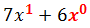Physical models like algebra tiles are one way to approach modeling the reason why we cannot combine 7x and 6. Let's look at another approach as to why we should not combine 7x and 6. Here's a contrived exchange between a student and a teacher that looks like how I approach this in my classroom verbally.

Teacher: What is the exponent on the x? <points to the x in 7x>

Student: Zero!

Teacher: If the exponent were a zero, we know any nonzero number to the zero power is 1. Then we would be multiplying the 7 by a 1, and we know multiplying any number by 1 does not affect the number's value. Also, we use zero to represent 'none.' So, if the exponent were a zero, then there would be 'no' x.

Student: Then the power must be a 1.

Teacher: That makes sense. What is the power of x on the 6? <points to 6>

Student: There's no x there!

Teacher: Would it be legal to draw a  ghost x0 to mean there is no x there?

Student: Okay.

Teacher: On page 89 of our algebra text, the author states "3x and 5x are like terms because they contain the same form of the variable x." The author then says 3x + 5x = 8x. What do you think the author means by 'same form'?

Student: The powers of x match in both.

Teacher: If that's true, then is it possible to combine 7x and 6 using addition or subtraction?

Student: No.

Then the curtains fall. The teacher and students move on to another problem. But let's take a second look. We still need a convincing argument to demonstrate the expressions 7x and 6 cannot be combined. Let's look at some specific cases.Suppose x = 1. Then the student is correct, since 7(1) + 6 = 13. But this substitution does not allow x to vary freely. Another way we can convince the student we cannot combine 7x and 6 would be to use a graph.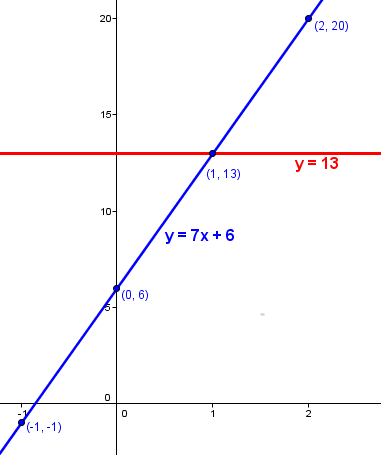The fact that the red line y = 13 and the blue line y = 7x + 6 is pretty convincing evidence the two quantities 13 and 7x + 6 are not the same for any x value other than 1, the intersection point on the graph. We can counsel the student that y = 13 is constant while y = 7x + 6, the line with slope 7 and y-intercept 6, depends on x. Let's consider another special case.

Suppose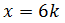for some integer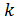. We can look at some specific cases to help our thinking about the consequences of this selection for x.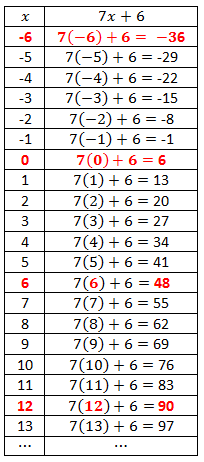We can now discuss the earlier statement, "Use the Distributive Property if needed." If we consider the case where x is an integer multiple of 6, then we have7k and 1 are not like terms, just as 7x and 6 are not like terms. 7k + 1 only equals 8 if k = 1. Why even think this way? Because we can tie the original problem back to factoring.I tell my students factoring is like playing tennis. Metaphorically speaking, we know a volley is over with in tennis based on what the ball does. We know we are 'done' factoring when we have an expression made of prime factors. I tell my students factoring is essentially a two step process:

Step 1. Greatest Common Factor (GCF)

Step 2. Depends on terms that remain. Applying a possible strategy may involve the whole collection of terms or a subset of the terms in the problem.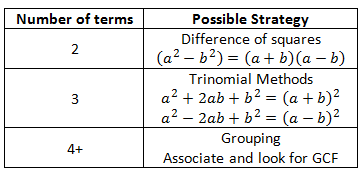We volley back and forth - do both steps - until we obtain expressions that are prime or constants that can be written as the product of primes. For instance, in the problem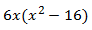, we could rewrite 6 as the product of 2 and 3... but the binomial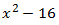can be rewritten as the product of prime linear factors x + 4 and x - 4.

Why do we consider x + 4 prime? Since the value of x varies, if we only consider integer values of x, then x + 4 may give a composite number or a prime number. Since we do not know the value of x, we think of x + 4 more conservatively as prime.

It does not take much to convince students that all numbers are divisible by 1. I insist my students look for a GCF every time, even in trivial problems, and identity the GCF is 1, or more specifically,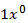. I try to emphasize that when we factor expressions with multiple terms, we look to factor out the "lowest" power the variable.

If we try and factor the original problem using this approach, we have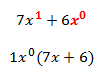since GCF(7, 6) = 1 and GCF(x, x^0) = x^0 = 1. This approach is particularly useful for later work in calculus, specifically when taking derivatives of expressions. My calculus students sometimes struggle with reconciling their solutions with the answer the text provides because they may not totally understand the factoring necessary for simplification. Students stumble when asked to find the GCF of the expression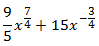. If students are accustomed to identifying the GCF, then problems of this type aren't as troublesome.

# Procedural Understanding in Teaching Mathematics

My self-imposed holiday blogging break is over. <cracks knuckles>The holiday break affords teachers the opportunity to reflect. One dimension I think many overlook in teacher reflection is to sit and think deeply about one's content area. I would even be bold enough to say the best math teachers are mathematicians at heart. When I sit down to think about mathematics, as a high school math teacher, I like to approach it like a person that can solve a Rubik's Cube. When you watch this feature below on a speed solver, Chester Liam makes the following claim about speed solving a Rubik's Cube:

As a speed solver? No, there is no math involved, no thinking involved. It's just finger dexterity and pattern recognition. There is nothing, no thinking involved in the entire solving process. [1:02 - 1:14]

We can unpackage Chester's thoughts about the Rubik's Cube and how he solves it so quickly by applying mathematical structure to the cube. However, we should ask: what is the objective? What are we trying to do? There's gobs and gobs of mathematics wrapped up in speed solving a Rubik's Cube, but if Chester were to pay attention to the procedures he is applying, it would inhibit his ability to solve the cube quickly.

But consider this: what if Chester attempts to teach how to solve the cube to another person? What would he have to do? What examples, explanations, and demonstrations would he utilize to teach his pupil speed solving? What implications does this thinking have on teaching mathematics? There would be many features of speed solving the learner may not perceive until Chester brings it to the learner's attention. And if Chester's choices are calculated, deliberate, purposeful... the learner may be helped or hindered dependent upon Chester's ability to communicate his thinking which leads to the automaticity of the procedures he applies to solve the cube. Just like learning how to read or learning how to drive a car, we want to teach learners how to do these tasks so well they become 'automated' at some level.  To understand mathematics deeply, I believe it is often necessary to unpackage some of these automated tasks. I will share an example of such a mental exercise below.

Today I've been thinking about procedures we accept as true while doing math at the high school level, algebra in particular. As an example, suppose we want to determine the location of the x-intercept for the line 5x - 3y = 7. We might approach this 'task' in the following way: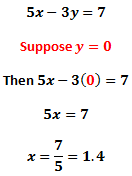And we might even graph the line to confirm our solution...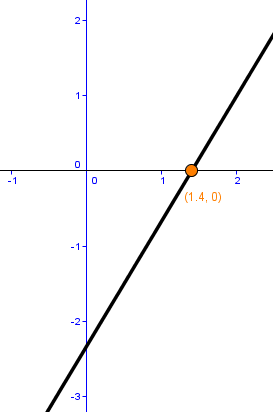Yep. There it is. The x-intercept at (1.4, 0). As a student, we might simply yawn and move on to the next exercise. The student must recognize the y-coordinate of the line will be zero when the line crosses the x-axis. Yes, we have a solution, but I'm not so sure as a math teacher I'm satisfied to stop there. What if we take a different approach? Let's turn the Rubik's Cube and look at the problem another way. A 'typical' algebra student might subscribe to the church of y = mx + b and do the following...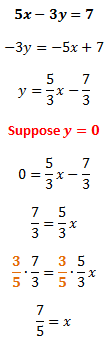Just another equally valid path to the value of the x-intercept. I'd like to focus on something in one of the above lines.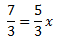This statement says five-thirds of some mystery number is seven-thirds. So, what's the mystery number? I think if we asked a room full of high school math teachers to draw a diagram explaining why we multiply each side of this equation by the multiplicative inverse of 5/3, namely 3/5, we would get some really surprising results. It's not an indictment of teacher education. Rather, it's to say some teachers may not have considered the how's and why's of this procedure before for the same reason someone learning to speed solve a Rubik's Cube may miss a key structure. They may not have the experience of needing to know why it works. Rather, it was more important that they can find the x-intercept of the line; the multiplication by the multiplicative inverse was viewed as "below" the task or level at hand. Perhaps a student never asked "why?" at the critical moment to give the teacher pause.

I struggled to produce the corresponding fraction diagram without trying to reverse engineer the solution. When I write this blog, I often worry I might make a mistake that will be indelibly written into the electronic space of the Internet. But this worry violates the spirit of my blog's theme: that we need to make mistakes in a good direction to evolve our mathematical understanding. Here's the images of my failed by-hand attempts to generate this fraction diagram:Chicken scratches, page 2

I had trouble thinking about making the diagram because 5/3 and 7/3 have the same denominator, so I wrote out some equivalent fractions. Then, I wanted to use "ninths" because that made sense to me in terms of the grid on the graph paper I had cut apart. But, I then realized I would need to cut fifths to find the mystery number, and I was REALLY struggling with trying to free-hand cut fifths with the grid in the background. That led me to coordinatize the points of the polygon. Then I had a problem with relationships between the linear units on the horizontal axis and the area (the fact the polygon is not "one" unit vertically... which is basically the notion of a unit fraction we see emphasized in CCSSM). So I abandoned the paper approach in favor of Geogebra because I could generate better precision and be more efficient with respect to time. <Sorry for the sloppiness of this paragraph, but it does describe my thinking and the mistakes I made.>

Below is an image of the fraction diagram I constructed using Geogebra.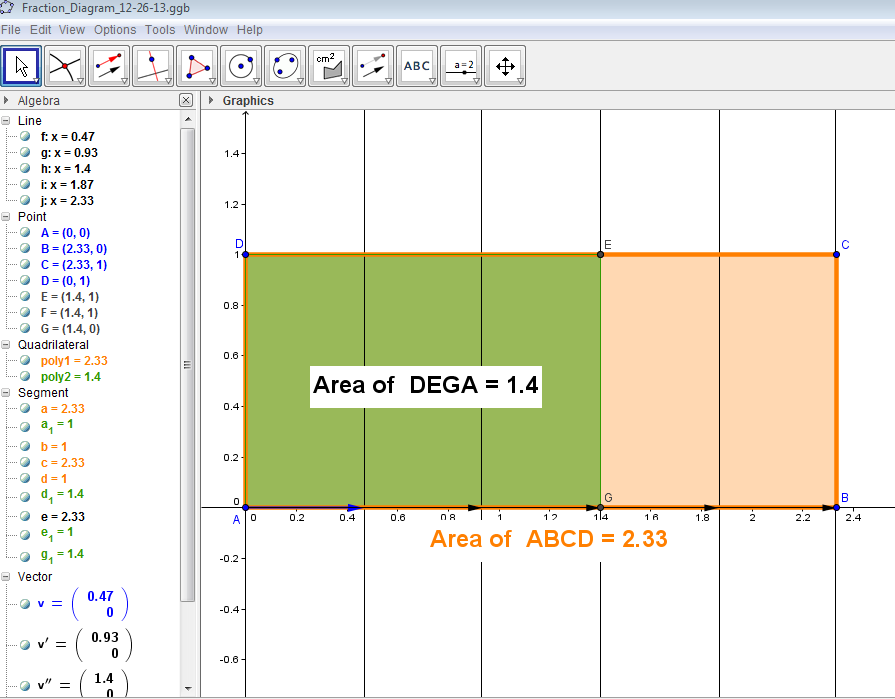When stating the equation, we should think of it as a verbal statement: "Seven-thirds is five-thirds of what mystery number?" Well, if the green polygon represents a whole, then the orange polygon is one-and-two-thirds of that whole. Then the green area of 1.4, which equals 7/5, corresponds to the solution. Mentally, how in the world did I end up with fifths, then? How did I know to cut the horizontal into fifths using vectors and vertical lines in the coordinate plane?

We can think of a fraction in the most basic way. Consider 5/3. If the denominator indicates the number of pieces we partition from a whole, and the numerator indicates how many pieces we possess, then I knew we needed to cut the orange rectangle in a way that would make five pieces. It gets to the root cause of WHY we invert and multiply. The numerator 5 becomes the desired number of pieces, considered in a denominator.

For the sake of time, I will stop my mental exercise there. Between working the problem, generating the fraction diagram by hand and on Geogebra, and typing this up, I've spent about two hours roughly on this article. This professional development is incredibly powerful for me as a teacher, and it's absolutely free (well, not quite free, I do pay for the website hosting, but you get the idea).

Let's end by stirring the discussion pot. Consider our understanding of how to find the x-intercept of the given line. Does our understanding, or lack of understanding, of the fraction diagram and how to construct the fraction diagram (essentially "invert and multiply" in many high school classrooms) inhibit our ability to solve the original problem? Is it still possible to understand the original solution without knowing all the nuts and bolts of the fraction procedure? Stephen Wolfram argues in favor of using computers to automate trivial computation procedures to help us access problems in the world outside school.  How will our teaching of mathematics change as computers continue to become faster and more powerful?

# Blue Cows & The Domain of a Function • 1 CommentSuppose for a moment a parent shows a child images of 10,000 blue cows. Yep. No typos. Ten. Thousand. Blue. Cows. We are talking blue. Like the cow pictured at the left. 10,000 is a healthy number of cows. This would amount to showing the child one blue cow every second for roughly 2 hours, 45 minutes. The child might conclude, given this overwhelming evidence, every cow is blue. We could really rock the child's world by introducing an image of a white cow or a brown cow or a black cow. This counterexample would stimulate the child to re-evaluate his or her conception about what makes a cow. The hope would be that the parent would help the child understand better the definition of "cow." What makes a cow? Four legs? Not necessarily, the cow could be an amputee. An udder? Not necessarily - couldn't the cow be a bull? Horns? A tail? A particular set of adenine, guanine, thymine, and cytosine? Do we need to be that specific? We collectively have a definition we use for the animal "cow," and it's often based on experience. As you are reading, you may have even conjured up, in your mind's eye, a picture of a cow or two. Specifically, that definition of "cow" may vary according to the context in which we are operating.

Take the above situation and replace every word "cow" with "mathematical example." If developing students with strong mathematical understanding is the goal, we must be wary of how to model for students how to behave when the white cow or brown cow or black cow comes along.

My experience with teachers and students tells me identifying the domain of a function is often tricky business for students. What types of functions does a typical Algebra 2 book examine when looking at the domain of a function? They look at lines, parabolas, and cubics for sure. A student sees the domain of a run-of-the-mill linear function and a run-of-the-mill cubic function are the same. They see the domain for a parabolic function is also the same. The student's mind attempts to search for some sort of pattern. The student may formulate misconceptions that do not generalize. It is a challenging task for the teacher to develop a robust understanding of domain in students. We hope the student understands the notion of a function, input values, binary operations that are not closed in the real number system, etc.

I was helping a former student yesterday with some material for an upcoming College Algebra exam. We came across the following problem.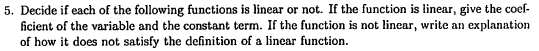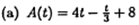There are many things to like about this question. The student must have a pretty solid understanding of what linear functions look like. The student must understand A(t) does not mean the product of quantities A and t. The student must recognize the quantity t/3 can be rewritten as (1/3)*t by leveraging the distributive property to combine the like terms 4t and t/3. The student must also recognize 8 can be rewritten as 8*t^0 to explain why 8 is not a like term with the others. Our work for the problem is below.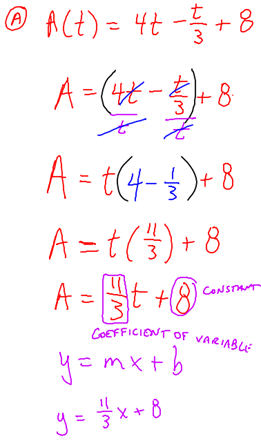No trouble, run of the mill example (blue cow).

Now for the brown cow.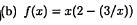What a GREAT QUESTION. <Trumpets herald from the heavens>

Here's some initial work on the problem, which looks an awful lot like the student that does not see the problem for what it is - something different.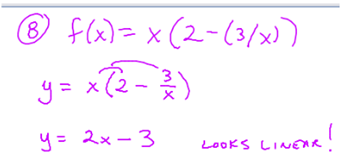As a teacher, I was thinking of how to leverage other connected ideas to help the student deepen his understanding of linear functions. Heck, we even graphed it in Geogebra and the silicon genie confirmed the student's suspicions about linearity with a picture.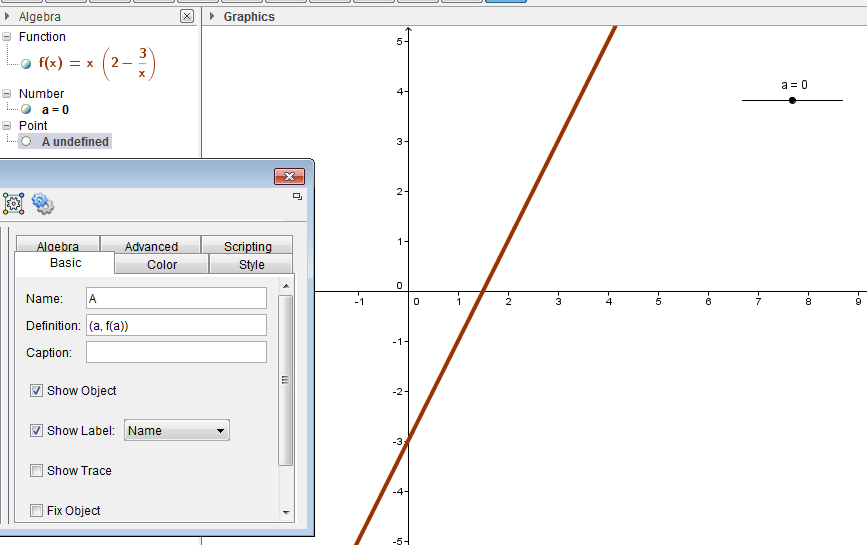I put all that other stuff in the sheet (the slider and the point A whose x-coordinate is governed by the value of the slider) after the fact. The student was convinced the function was linear and was ready to move on.

My background knowledge from modern algebra and rings and binary operations and all that jazz let me see the problem for what it was. I was trying to think through how to meet the student at his level to help him develop an understanding that would allow him to identify functions that may appear linear but have potential domain issues. One could argue the function is "linear" everywhere except at x = 0. I asked the student about the operations he saw going on within the original function statement.Multiplication by x, the difference between 2 and the quantity 3/x, and the quotient of 3 and x. The following exchange ensued.

Mr. A: "What do you get when you add two real numbers?"
Student: "A real number."
Mr. A: "What happens when you subtract two real numbers? What do you get?"
Student: "A real number."
Mr. A: "What happens when you multiply two real numbers?"
Student: "You get a real number."
Mr. A: "So what do you think about division?"
Student: "You get a real number."
Mr. A (with poker face): "Is what you said always true? Will there ever be a case where you divide two real numbers and you do not obtain a real number as a result?"

Then we did a little work to back it up.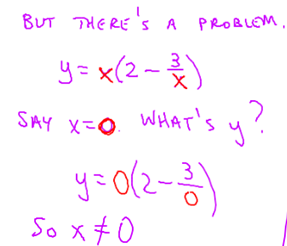I even went so far as to write this statement on the board: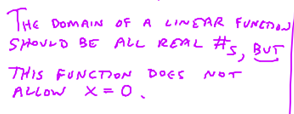The student immediately questioned this claim, since we had worked this problem earlier: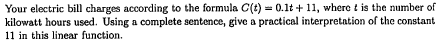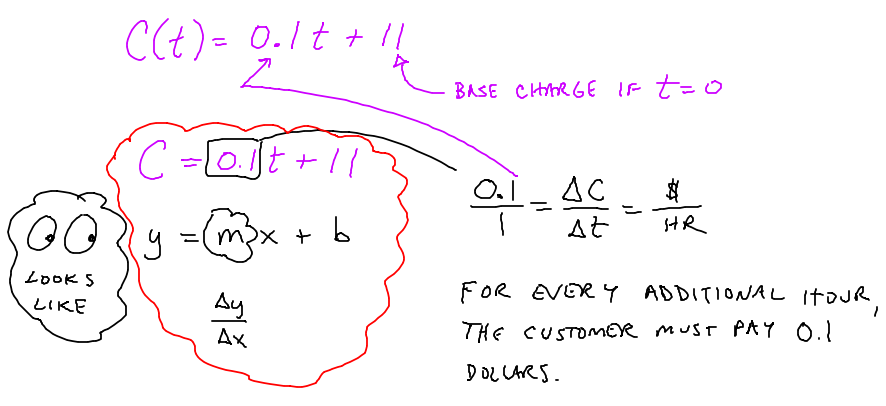We had mutually agreed the domain of t in the function above would be numbers greater than or equal to zero, since it wouldn't make sense for the variable t to take on negative values, as this would correspond to times before the billing cycle began. From the perspective of polynomials, however, the function C(t) = 0.1t + 11 has a domain of all real numbers. We choose to impose the restriction that t must be strictly greater than or equal to zero in the context of the problem. The student was drawing from a previous experience in trying to understand the domain issue we faced. The fact we disallowed negative values appeared to be a contradiction to the student because the purple sentence I wrote contained the phrase, "does not allow."

My thoughts turned to polynomials and how I could help the student move forward. I asked the student, "What is the definition of a polynomial?" My hope was that he would respond with something about the operations allowed on the variable. No such luck. After a long pause, I said, "Well, let's do what any sensible person would do. Let's look it up."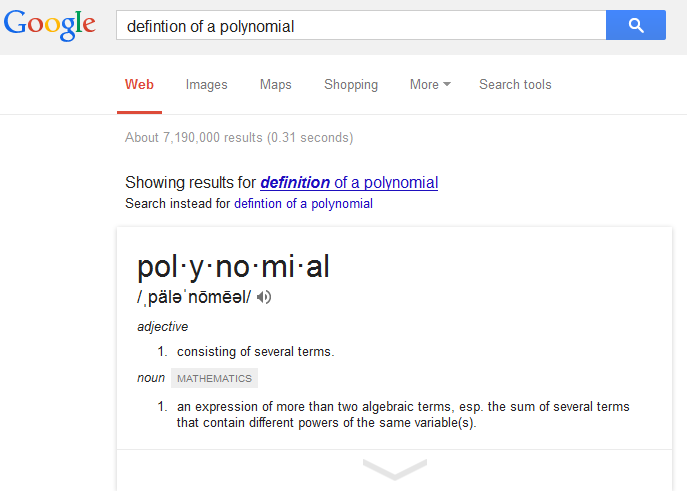This definition didn't help unmuddy the waters. I am a firm believer in not clicking on the first link in Google immediately and helping the student reason through which links to use. After some digging, we eventually clicked the first link and came across this definition: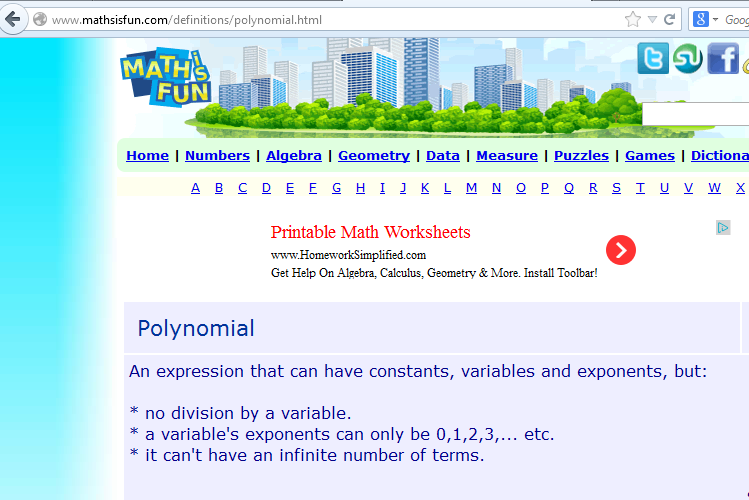Indirectly, this definition states the operations addition, subtraction, and multiplication on the variable are permitted. I wanted the student to also recognize there are different classifications into which functions could fall, like rational functions or radical functions.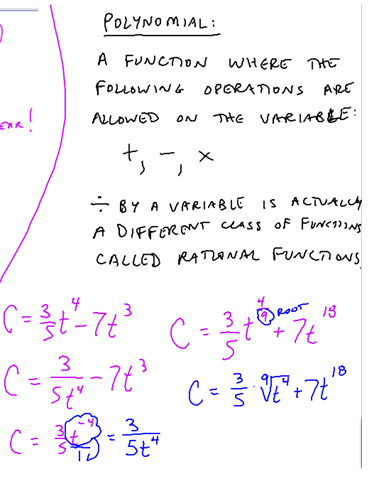We used the examples towards the bottom to address different possibilities for powers that may lead to division by a variable or taking the root of a variable. This rich discussion took roughly forty-five minutes to run its course, with some pauses for questioning, thinking, and doing some algebra. I finished our discussion of this problem with some other looks this problem might present.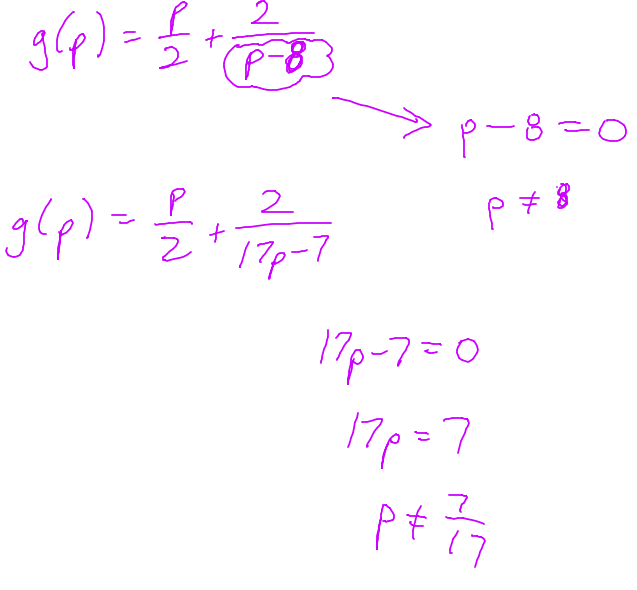I hope you enjoyed this article. I would argue one of the fundamental purposes of schooling in mathematics should be to help students develop a rich understanding and to know how to behave when a cow of a different color comes along.

# (Not So Typical) Dice Problems • 1 Comment

Probability and counting questions associated with rolling two dice are frequent at math contests. For example, one might ask, "What is the most common sum when two dice are rolled?" The grid below helps the student see the most common sum.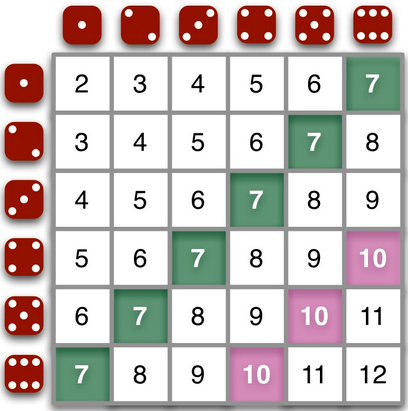Exhibit A: The sums of two dice. (Ignore the pink stuff; 7 is the most common sum).

Suppose we construct the probability distribution for rolling two dice.Exhibit B: Probability simulation for rolling two dice. 7 is the most likely sum.

One of my colleagues has 11 dice in her classroom. This made me wonder what would be the most likely sum for 11 dice. How would we go about answering this question?

Problems like this one show students the value of breaking a larger problem into smaller problems. Let's construct a table for the minimum and maximum sums for rolling n number of dice and see if we can determine some patterns. Our aim is to generalize our findings to n dice.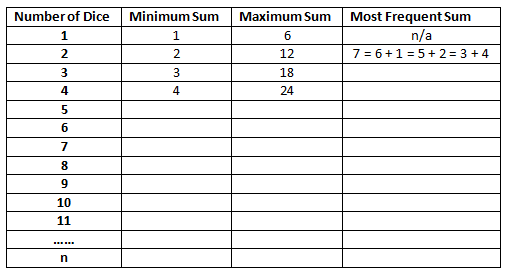Exhibit C: A preliminary table for rolling n dice.

How would you support students in working towards the generalization? For example, modeling two dice with a table is fairly simple, as shown above. What about modeling three dice? Sure, you could go three dimensional... but what about four dice? Five dice? This problem poses some great modeling questions.Exhibit D: Polynomial approach to obtaining dice counts for the case where n = 2.

# The Quadratic Formula Seen on a Graph

I am a supporter of the Common Core State Standards for Mathematics (CCSSM). While far from perfect, I think the CCSSM Mathematical Practices are downright beautiful. I see shades of these practices in my classroom from time to time. I am striving to develop these behaviors, like constructing viable arguments and critiquing the reasoning of others, in all of my students.

While reading through the discussion on Algebra (page 62 of the CCSSM document) earlier today, I found the following:

An equation is a statement of equality between two expressions, often viewed as a question asking for which values of the variables the expressions on either side are in fact equal. These values are the solutions to the equation. An identity, in contrast, is true for all values of the variables; identities are often developed by rewriting an expression in an equivalent form...

...The same solution techniques used to solve equations can be used to rearrange formulas. For example, the formula for the area of a trapezoid, A = ((b_1+b_2)/2)h, can be solved for h using the same deductive process.

Rewriting an expression can often provide greater understanding of its underlying structure. As a student, I had a rough experience with geometry in high school; however, I did very well in algebra class. Dynamic software provides a learner like me the opportunity to see the connections between algebra and geometry. I can bootstrap my way up to better geometric understanding by seeing the connections between symbolic manipulation and graphs.

Below is an image of a Geogebra sheet I made which demonstrates how we can rewrite the quadratic formula as the axis of symmetry of the parabola plus or minus a distance.This sheet demonstrates the graphical connection between the quadratic formula and the roots. Symbolically, we can rewrite the quadratic formula as two fractions with denominator 2a.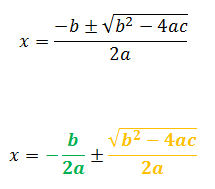The first fraction, the green -b/(2a), gives the axis of symmetry of the parabola. The second orange fraction gives the distance we walk to the left and right of the axis of symmetry. We can think of an x-intercept in the following way: start at the axis of symmetry, then walk the same distance (the second fraction) in the positive or negative direction along x.

If this discriminant b^2-4ac is positive (the radical stuff), there are two distinct real roots.
If this discriminant is zero, there is one repeated real root.
If this discriminant is negative, then there are two imaginary roots. Graphically, there are no real solutions, demonstrated by the Geogebra sheet.

This approach demonstrates visually the utility of the discriminant in determining the number of roots of a parabola written in standard form.

# Math Club T-Shirt Design: Bearcat Head

I love to see the creativity of my students realized.

Below is our Math Club t-shirt design we will proudly sport at UNL Math Day next week.Exhibit A: Original image of our school mascot, the Scottsbluff BearcatExhibit B: The piecewise function which graphs the outline of the Bearcat head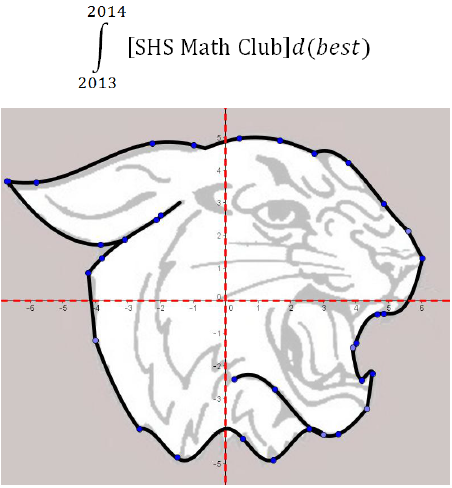Exhibit C: Finished design for the front of the shirt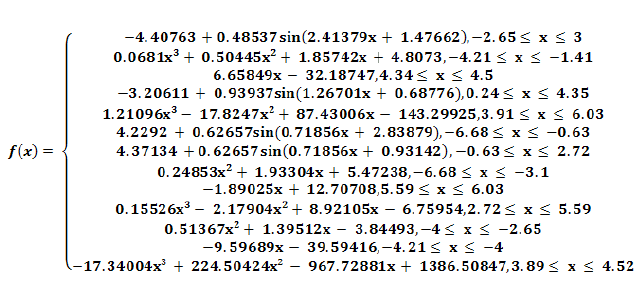Exhibit D: Finished design for the back of the shirt

# Geometry Week Day 4: y = mx + b, the x-Intercept, and Bowl Contest Problems • 1 Comment

Gesturing is a powerful way for students to learn. I have students gesture with their hands often, particularly when we examine behavior of graphs. One of our teachers was working to help students remember the slope-intercept form of a line. She found this video on Youtube:

The teacher had the students do the gestures to help remember the parts of the slope-intercept form. I wanted to help the students understand the graphical effects of changing the values of m and b on the line y = mx + b. I started with a blank Geogebra sheet and constructed the graph of y = mx + b actively in front of the students.Students had no trouble with the effect altering the value of m had on the graph. However, something interesting happened when I asked the class, "What is your prediction for the effect changing b will have on the graph?" One of the students quickly replied, "The black point will move along the x-axis." I think my face betrayed my attempt to remain neutral. I said with my mouth, "You're right, the black point will move along the x-axis. What else will happen?" The students eventually pointed out the relationship between the b-value on the slider and the y-coordinate of the pink point.

But that student's comment has stuck with me since yesterday. I hadn't really considered before how we would go about defining the movement of the black point along the x-axis.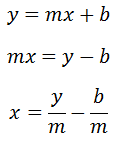Let's take a second look at the graph.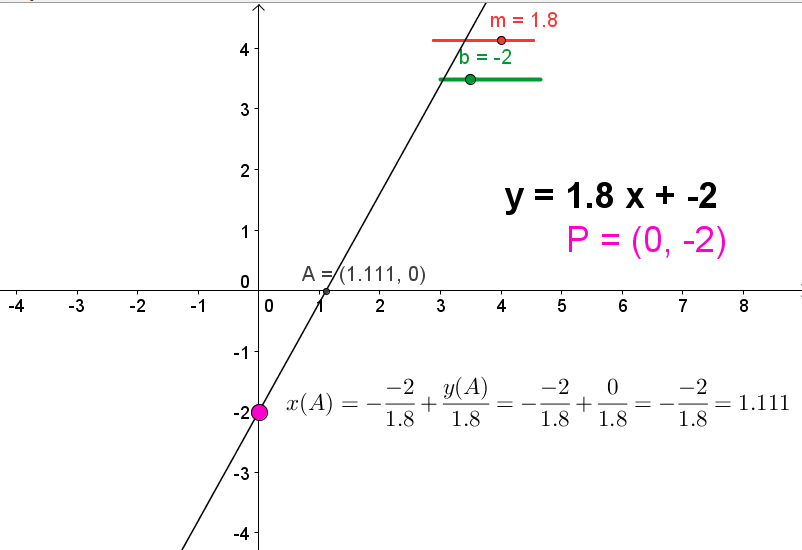We can define the x-coordinate at point A using x = (y/m) - (b/m). I defined the text box using the LaTEX editor in Geogebra.